Courses

# Test: Plastic Analysis - 2

## 15 Questions MCQ Test Topicwise Question Bank for Civil Engineering | Test: Plastic Analysis - 2

Description
This mock test of Test: Plastic Analysis - 2 for Civil Engineering (CE) helps you for every Civil Engineering (CE) entrance exam. This contains 15 Multiple Choice Questions for Civil Engineering (CE) Test: Plastic Analysis - 2 (mcq) to study with solutions a complete question bank. The solved questions answers in this Test: Plastic Analysis - 2 quiz give you a good mix of easy questions and tough questions. Civil Engineering (CE) students definitely take this Test: Plastic Analysis - 2 exercise for a better result in the exam. You can find other Test: Plastic Analysis - 2 extra questions, long questions & short questions for Civil Engineering (CE) on EduRev as well by searching above.
QUESTION: 1

### A propped cantilever beam of uniform moment capacity M0 is shown in figure below.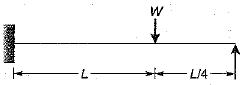What is the collapse load W?

Solution:

Number of plastic hinges required for complete collapse - r + 1
= (3 - 2) + 1 = 2
The plastic hinges will be formed under the load and at the fixed end of the beam respectively.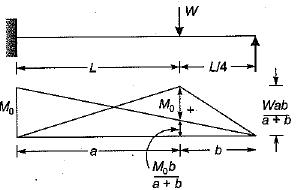But a = L and b = L/4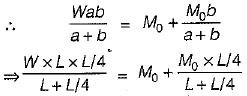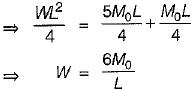QUESTION: 2

### A prismatic beam (shape factor, \$) fixed at both ends carries UDL throughout the span. What is the ratio of collapse load to yield load?

Solution:

The collapse load for a prismatic beam fixed at both ends and caries UDL throughout the span is given by,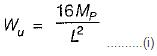where Mp is its plastic moment.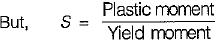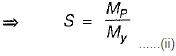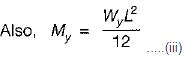Substituting value of S from (ii) in (iii), we get,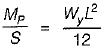Substituting value of Mp from (i), we get,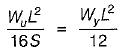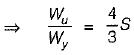QUESTION: 3

### A simply supported beam of uniform cross-section has span L and is loaded by a point load P at its mid-span. What is the length of the elastoplastic zone of the plastic hinge?

Solution:
QUESTION: 4

In a T-section shown in figure below, what is the distance of plastic neutral axis as measured down from top?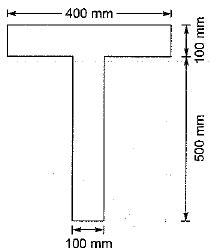Solution:

Total area, A = 400 x 100 + 100 x 500
= 90000 mm2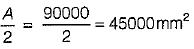Thus the plastic neutral axis lies outside the flange because the flange area is only 40000 mm2.
Let the plastic neutral axis lies y mm below the junction of flange and web.
∴ y x 100 = 45000 - 40000
⇒ y = 50 mm
∴ Plastic neutral axis as measured from top of flange = 100 + y
= 100 + 50 = 150 mm

QUESTION: 5

At the location of a plastic hinge,

Solution:
QUESTION: 6

The mechanism method and the statical methods give

Solution:

The static method represents the lower limit to the true ultimate load and has a maximum factor of safety.

QUESTION: 7

Arrange the following cross-sections in the increasing order of their respective shape factors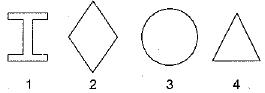Solution: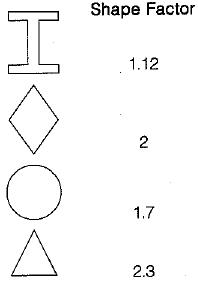QUESTION: 8

Position of plastic hinge in the beam shown in figure subjected to collapse ioad will be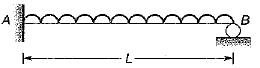Solution:

Because maximum bending moment develops at end A and between mid span and end B.

QUESTION: 9

The plastic hinge formed in a collapse mechanism are 4 and the indeterminacy is 3. The collapse is

Solution:

If the number of plastic hinges in the collapse mechanism are less than (r + 1) the collapse is called partial collapse. In such a case, part of the structure may fail making it useless as a whole. If the number of plastic hinges in the collapse mechanism are (r + 1) the collapse is called complete collapse. Such a mechanism has only one degree of freedom. If the number of plastic hinges developed are more than (r + 1), the collapse is called over complete collapse. In such a case there are two or more mechanisms for which the corresponding value of the load is the same, this load value being the actual coljapse load.
If the degree of indeterminacy is r, and the number of plastic hinges developed is N then,
N < (r + 1) Partial collapse
N = r + 1 Complete collapse .
N > r + 1 Overcomplete collapse

QUESTION: 10

The effect of axial force and shear force on the plastic moment capacity of a section are

Solution:
QUESTION: 11

The plastic section modulus for a rectangular section of width b and depth d is

Solution:
QUESTION: 12

Which of the following conditions is to be satisfied both in elastic and plastic analysis?

Solution:
QUESTION: 13

Consider the following assumptions made in the plastic theory
1. Distribution of strain across the section is linear
2. There is an axis of symmetry in the cross- section
3. The influence of normal and shearing forces is neglected
4. Strain energy stored due to elastic bending is ignored

Which of these assumptions are correct?

Solution:
QUESTION: 14

A continuous beam is loaded as shown in the figure below. Assuming a plastic moment capacity equal to Mp, the minimum load at which the beam would collapse is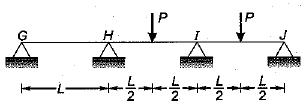Solution: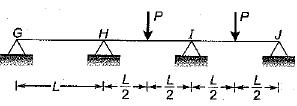For collapse in IJ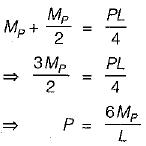For collapse in HI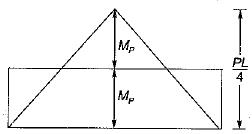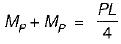⇒ P = 8MP/L
∴ Minimum load for collapse = 6MP/L

QUESTION: 15

For an I-beam, shape factor is 1.12. The factor of safety in bending is 1.5. if the allowable stress is increased by 20% for wind and earthquake loads, then the load factor is

Solution: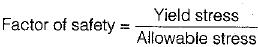With increase in allowable stress, the factor of safety will reduce to 1.5/1.2 = 1.25.
∴ Load Factor = FS x shape factor
= 1.25 x 1.12
= 1.40